×

Search anything:

# Iteration Method for Time Complexity

#### Time Complexity AlgorithmsGet this book -> Problems on Array: For Interviews and Competitive Programming

In the following article, we have presented the Iteration method for finding the Time complexity of an algorithm in detail.

1. Introduction to Recurrence relations
2. Iteration Method
3. MCQs on Recurrence relations

Pre-requisites:

# Introduction to Recurrence relations

Recurrence relation is way of determining the running time of a recursive algorithm or program. It's a equation or a inequality that describes a functions in terms of its values and smaller inputs. Now before jumping on to various methods of solving recurrence relation, let's first take a look at the example of recurrence relation.
Let us consider T(n) to be the running time of a given problems on size n, the problem in our case will be finding the nth fibonacci number. Let F(n) denote the nth fibonacci number, therefore F(n) = F(n-1) + F(n-2) and T(0) = a , a is a constant.

The recurrence relation will be :
T(n) = c + T(n-1), where c is a constant

There are mainly four methods of solving recurrence relation :

1. Iteration method
2. Substitution method
3. Master method
4. Recursion tree method

In this article our primary focus is Solving recurrence relation via Iteration method, hence we will deep dive into the process through examples and explanations.

# Iteration Method

Iteration method can be also be called a Brute force method because we have to substitute the recurrent part value until a pattern is observed, thereafter we use mathematical summation technique is used to find the recurrence.

Now let us take an example :

Recurrence relation : T(1) = theta(1) and T(n) = n^3 + 2T(n/2)
Solution :
T(n) n^3 + 2(T(n/2)) ------------ (i)

On putting value of T(n/2) in (i) we get :

T(n) = n^3 + 2((n/2)^3 + 2(T(n/4))) ------------ (ii)

Putting value of T(n/4) in (ii) we get :

T(n) = n^3 + 2((n/2)^3 + 2((n/4)^3 + 2T(n/8))))

Using similar subtitutions further we get :

T(n) = n^3 + 2((n/2)^3 + 2((n/4)^3 + 2((n/8)^3 + 2(T(n/16)))))

We notice that n^3 term is replaced by (n/2)^3, then by (n/4)^3 and so on ............

Now let's simply it further :

T(n) = n^3 + 2(n/2)^3 + 4((n/4)^3 + 2((n/8)^3 + 2((T(n/16))))
T(n) = n^3 + 2(n/2)^3 + 4(n/4)^3 + 8((n/8)^3 + 2(T(n/16)))
T(n) = n^3 + ((n^3)/4) + ((n^3)/4^2) + ((n^3)/4^3) + 16(T(n/16))

Now form the original problem we know that :
T(1) = theta(1)
hence,
T(n/2^i) = 1 when i = log n (base 2)
therefore
T(n/2^4) = 1 when 4 = log n (base 2)

T(n) = n^3(1 + 1/4^1 + ------------ + 1/4^i-1) + 2^i(T(n/2^i))

Now we know that i = log n (base 2)

T(n) = n^3(1/4^0 + 1/4^1 + --------- + 1/4^i-1) + 2^i(T(n/2^i))

On simplifying further we get:

T(n) = n^3(1/4^0 + 1/4^1 + ----------- + 1/4^i-1) + n*T(1)

Now n(T(1)) becomes theta(n), we also have finite G.P. with first term equal to 1 and common ratio equal to 1/4, lets apply the sum formula for finding the sum of the terms of finite G.P.

S(n) = a(1 - r^n)/(1 - r) is the sum of first n terms of G.P.

T(n) = theta(n) + n^3(1(1 - n^i-1)/(1-1/4))

Since its a finite G.P. hence finite time will be taken to execute

T(n) = theta(n) + n^3(theta(1))
T(n) = theta(n) + theta(n^3)
T(n) = theta(n^3) Ans

# MCQs on Recurrence relations

(i) Find the solution of given recurrence relation F(n) = 20F(n-1) - 25F(n-2), where F(0) = 4 and F(1) = 14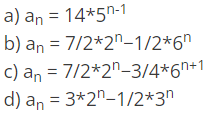Ans : b

Explanation : First find the characteristic polynomial i.e. x^2-20x+36=0 in this case, having found the roots find the form of recurrence equation i.e. an=a(2^n)+b(18^n), find the value of a and b using equations F(0) = 4 and F(1) = 14 i.e. a=7/2 and b=-1/2, hence the answer is option b.

(ii) Determine the recurrence relation for the following series 1,7,31,127,499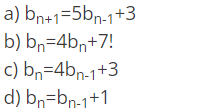Ans : c
Explanation : On observing carefully, we see that the difference between the consecutive terms is a factor of 4, therefore 1.4=4,7.4=28,31.4=124 so on, ending term is 3 less than the next term therefore the answer will be option c.

(iii) Find the solution of given recurrence relation F(n) = 6T(n-1) - 8T(n-2), given that T(0) = 3 and T(1) = 5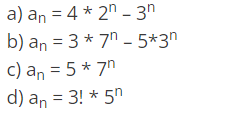Ans : b
Explanation : Follow similar steps as mentioned in the first problem.

(iv) Find the solution of the recurrence relation T(n) = 5T(n-1) + 6T(n-2)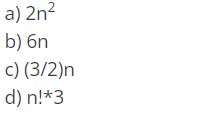Ans : b
Explanation : Just check on the left side of the given equation for all the options individually, we find that 6n satisfies the equation hence answer is option b.

(v) Find the value of T(2) for the recurrence relation T(n) = 17T(n-1) + 30n, given that T(0) = 3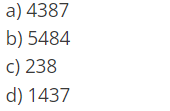Ans : d
Explanation : First calculate the value for T(1) i.e. T(1) = 17T(0) + 30 = 81, now calculate value of T(2) by putting the value of T(1) in it i.e. T(2) = 17T(1) + 60 = 1437, that is our required answer.

Thank You!

With this article at OpenGenus, you must have a strong idea of Iteration Method to find Time Complexity of different algorithms.#### Suraj Kumar

Developer and Open Source Enthusiast!

Iteration Method for Time Complexity